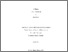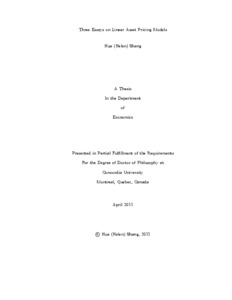Title:

Three Essays on Linear Asset Pricing Models

Shang, Hua (2011) Three Essays on Linear Asset Pricing Models. PhD thesis, Concordia University.Preview
Text (application/pdf)
Shang_PhD_F2011.pdf - Accepted Version
687kB

Abstract

This disertation includes three essays on linear asset pricing models.
The first chapter is concerned with the effects of including a low-variance factor which leads to a small signal-to-noise ratio in an asset pricing model. We rely on local asymptotics and define the low-variance as local-to-zero by being inversely related to the sample size. When a low-variance factor is present, the commonly applied Fama-Macbeth two-pass regression procedure yields misleading results. Local asymptotic analysis and simulation evidence indicate that the beta of the low-variance factor, risk premiums corresponding to all factors and the magnitude of associated variances are all unreliably estimated. Moreover, t- and F- statistics are unable to detect whether risk premiums are significantly different from zero. Additional simulation results also reveal that Kleibergen's statistic has some ability to detect the usefulness of different factors.
In the second chapter, I investigate the finite sample properties of the two-pass regression, the t-statistic, statistics proposed by Kleibergen (2009) and the specification tests when the first-pass regression slope coefficients --- betas --- are large, small and zero. In particular, I explore the effect of the number of assets on the properties of the statistics. The results reveal that most of the statistics tend to reach a conclusion that the factor should be included in the model or the model is correct more often that it should, especially when betas are small and the number of assets is large. The diagnosis of the results shows that the source of the problem lies in the large bias of the estimated risk premiums and the poor estimation of the variance-covariance matrix of the error terms in the first-pass regression.
The third chapter explores an economic explanation of commodity prices by considering the macro-economic exposure of commodity returns. Through estimating the stochastic discount factor representation of the linear asset pricing model, I find that investors are compensated for exchange-rate risk. The result is robust to different estimation methods, to different data sets and over longer periods of time.

Divisions: Concordia University > Faculty of Arts and Science > Economics Thesis (PhD) Shang, Hua Concordia University Ph. D. Economics 9 June 2011 Gospodinov, Nikolay 7641 HUA SHANG 22 Nov 2011 13:26 18 Jan 2018 17:31
All items in Spectrum are protected by copyright, with all rights reserved. The use of items is governed by Spectrum's terms of access.

Repository Staff Only: item control page

Back to top